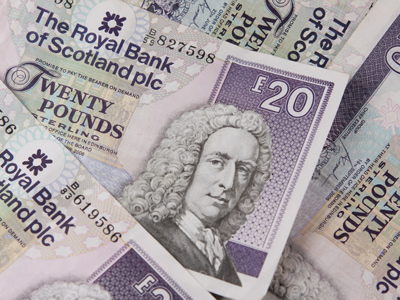What is 1/8 of £20.00?

# Level 5-6 Numbers - Fractions - Addition and Subtraction

Addition and subtraction - what could be easier? But you know that KS3 Maths is anything but easy! In this quiz you'll be adding and subtracting fractions.

Adding and subtracting fractions is not as difficult as it at first appears. All that you have to do is find their lowest common multiple and give both fractions this denominator. You can only add and subtract fractions if they have the same denominator - that's the number below the line. Fractions are made up of two numbers - numerators (the top or first number) and denominators (the bottom or second number). So, to add 25 and 23 you need to convert both fractions into 15ths. 25 = 615 and 23 = 1015. 615 + 1015 = 1615 or 1115. Simple when you know how!

Try the following quiz and you'll soon get the hang of adding and subtracting fractions. Take your time and consider your answers carefully. Good luck!

1.
What is the lowest common multiple of the denominators in 3/4 - 1/5?
9
12
18
20
It is the smallest number in the 4 and 5 times table
2.
How many sixths are there in 323?
12
22
32
42
2/3 is the same as 4/6
3.
What is 3/4 - 1/5?
1/20
5/20
11/20
15/20
20 is the lowest common multiple of 4 and 5 so we must convert both fractions to 20ths
4.
£6.00 = 1/8 of .......
£48.00
£52.00
£56.00
£62.00
Just multiply £6.00 by 8
5.
What is 3/5 - 1/4?
4/10
4/20
7/10
7/20
20 is the lowest common multiple of 4 and 5 so we convert both fractions to 20ths
6.
What is 1/8 of £20.00?
80p
£1.20
£1.25
£2.50
1/8 of £20.00 is the same as 1/4 of £10.00
7.
What is 1/4 + 1/2?
1/8
2/6
3/4
4/9
4 is the lowest common multiple of the denominators so we can convert 1/2 to 2/4
8.
How many sixths are there in 356?
15
17
23
27
There are 18/6 in 3 plus the remaining 5/6 makes 23
9.
What is the lowest common multiple of the denominators in 1/4 + 1/2?
1
2
3
4
4 is the lowest common multiple of 4 and 2, therefore change 1/2 into an equivalent fraction with a denominator of 4
10.
For the calculation 1/4 + 1/2, what would you change 1/2 into in order to find the answer?
3/4
2/4
1/4
4/6
The lowest common multiple is 4, and 2/4 is an equivalent fraction of 1/2